##### The Japanese Bonsai specialist
Direct order Contact Help / Services Newsletter# Lanterne granit zendoji gata130 cm.

› Lanterns, basins and statues › Stone lantern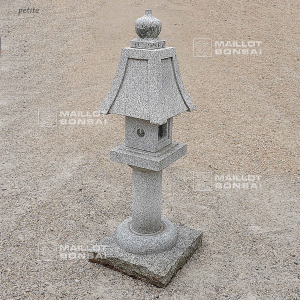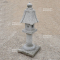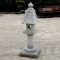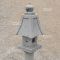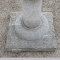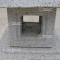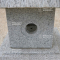ref. : 11995

885,00

voluminous/heavy item extra shipping of 75,00

Available quantity : 1Order

###### Description

Height: 130 cm
Top section: 35*35 cm Height of the column: +- 35 cm.

Diameter of the column: 15 cm.

Thickness: 40 cm

Weight: 150 kilograms

Dimensions of the square cage: 17*17 cm.

Height of the cage: 17 cm.

Dimensions of the pedestal: 45*45 cm.

Thickness : 14 cm.

An element like a lotus flower bud is on the top of the hat of the lantern which Height is 18 cm. Delivered in 6 elements. A Japanese nishinoya toro or lantern made of solid granite is notable for the elegant roof line and crown shaped like a lotus bud. decorates the central table light compartment featuring a flower stencil window and pierced holes. A bulb can be added to illuminate it, lighting pathways and entrances. The granite surface type is pineapple grain. Our garden elements (basins, granite lanterns, stones, and Japanese cobblestones) are usually stored outside. Indeed these elements may present patina and an ancient aspect (lichen and moss), which give them an additional authenticity charm. This aspect is commonly really appreciated in the traditional Japanese garden sphere. This model, called zendoji gata is little common and it is rare to find it in Japan. Guy Maillot chose to introduce it to his customers. The last picture is a scenery suggestion.

#lantern 4.7 #height 4.6 #elements 3.8 #japanese 3.8 #granite 3.7 #zendoji 3.7 #dimensions 3 #thickness 2.9 #lanterne 2.8 #gata130 2.7

###### Technical description
 Height 100-200 cm

Formule
(( ROUND((CHAR_LENGTH(b.article_nom)-CHAR_LENGTH(REPLACE(b.article_nom, 'height', '')))/LENGTH('height')) + ROUND((CHAR_LENGTH(b.article_description)-CHAR_LENGTH(REPLACE(b.article_description, 'height', '')))/LENGTH('height')) ) * 4.6) + (( ROUND((CHAR_LENGTH(b.article_nom)-CHAR_LENGTH(REPLACE(b.article_nom, 'elements', '')))/LENGTH('elements')) + ROUND((CHAR_LENGTH(b.article_description)-CHAR_LENGTH(REPLACE(b.article_description, 'elements', '')))/LENGTH('elements')) ) * 3.8) + (( ROUND((CHAR_LENGTH(b.article_nom)-CHAR_LENGTH(REPLACE(b.article_nom, 'japanese', '')))/LENGTH('japanese')) + ROUND((CHAR_LENGTH(b.article_description)-CHAR_LENGTH(REPLACE(b.article_description, 'japanese', '')))/LENGTH('japanese')) ) * 3.8) + (( ROUND((CHAR_LENGTH(b.article_nom)-CHAR_LENGTH(REPLACE(b.article_nom, 'granite', '')))/LENGTH('granite')) + ROUND((CHAR_LENGTH(b.article_description)-CHAR_LENGTH(REPLACE(b.article_description, 'granite', '')))/LENGTH('granite')) ) * 3.7) + (( ROUND((CHAR_LENGTH(b.article_nom)-CHAR_LENGTH(REPLACE(b.article_nom, 'zendoji', '')))/LENGTH('zendoji')) + ROUND((CHAR_LENGTH(b.article_description)-CHAR_LENGTH(REPLACE(b.article_description, 'zendoji', '')))/LENGTH('zendoji')) ) * 3.7) + (( ROUND((CHAR_LENGTH(b.article_nom)-CHAR_LENGTH(REPLACE(b.article_nom, 'dimensions', '')))/LENGTH('dimensions')) + ROUND((CHAR_LENGTH(b.article_description)-CHAR_LENGTH(REPLACE(b.article_description, 'dimensions', '')))/LENGTH('dimensions')) ) * 3) + (( ROUND((CHAR_LENGTH(b.article_nom)-CHAR_LENGTH(REPLACE(b.article_nom, 'thickness', '')))/LENGTH('thickness')) + ROUND((CHAR_LENGTH(b.article_description)-CHAR_LENGTH(REPLACE(b.article_description, 'thickness', '')))/LENGTH('thickness')) ) * 2.9) + (( ROUND((CHAR_LENGTH(b.article_nom)-CHAR_LENGTH(REPLACE(b.article_nom, 'lanterne', '')))/LENGTH('lanterne')) + ROUND((CHAR_LENGTH(b.article_description)-CHAR_LENGTH(REPLACE(b.article_description, 'lanterne', '')))/LENGTH('lanterne')) ) * 2.8) + (( ROUND((CHAR_LENGTH(b.article_nom)-CHAR_LENGTH(REPLACE(b.article_nom, 'lantern', '')))/LENGTH('lantern')) + ROUND((CHAR_LENGTH(b.article_description)-CHAR_LENGTH(REPLACE(b.article_description, 'lantern', '')))/LENGTH('lantern')) ) * 2.7) + (( ROUND((CHAR_LENGTH(b.article_nom)-CHAR_LENGTH(REPLACE(b.article_nom, 'gata130', '')))/LENGTH('gata130')) + ROUND((CHAR_LENGTH(b.article_description)-CHAR_LENGTH(REPLACE(b.article_description, 'gata130', '')))/LENGTH('gata130')) ) * 2.7)

## Secure payment## Delivery

Our logistic partners :04 74 55 23 48
Pépinière MAILLOT-BONSAÏ
Le Bois Frazy
01990 RELEVANT - FRANCE
on appointment